# Solving Equations

A typical maths textbook presents equation solving in a very ordered, structured way. Starting with one-step equations such as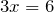, they introduce the single step solution process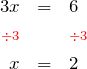before moving onto two-step equations such as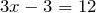. For these equations the solution proceeds in two steps as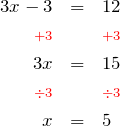and so on to equations with the unknown on both sides, equations involving fractions, equations with brackets etc. Each type of equation gets its own topic and lesson.

Now this may seem sensible, and certainly introduces all the key concepts, but in my opinion the approach is significantly flawed. It simply doesn’t teach the underlying, unifying concept of the solution to an equation well. If it did, the topic would not need to be taught and retaught, year after year, in essentially the same way. But so many students struggle to progress along the path of increasingly complicated types of equation, because this approach makes solving equations seem like a mass of subtly different and confusing rules, which further leads to the impression that there is really something fundamentally different between each of these “types” of equation. There is not! They are all exactly the same thing. If you can solve one, you can solve them all without having to know any more maths or any new rules. This will be recurring theme in this blog, and is what I will discuss below.

## All equations can be solved in the same way

Let’s stop and think about what an equation really is. It is a statement that two algebraic expressions, each of which is some mixture of numbers, pronumerals, and operators (i.e.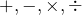and others), are equal. To solve an equation, we need to reduce this mixed up mess to a simple statement of the value of the unknown.

But how do you untangle a mathematical mess like, for example,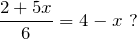Well, how would you untangle a tangled up garden hose? By reversing the tangling — doing the opposite. If it’s twisted to the left, twist to the right; if it’s wrapped around clockwise, unwrap it anti-clockwise. It might surprise you to know, an equation is just like that hose. Do the opposite, untangle the mess, and voilà — you will have a solution!

But what is the opposite for an algebraic expression? That’s easy, and the approach is the same no matter what type of equation you are solving. Mathematical operators come in opposing pairs: + and − are opposites, each undoes what the other does. Similarly,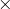andare opposing pairs. So if an expression is tangled by a, i.e. aoperator is keeping numbers and proumerals mixed up together on the same side of the equation, untangle by using a.

This is the first step: Untangle the expressions by using the opposite operator.

And how do you make sure your untangling is not changing the solution? Again, no matter what type of equation you have, it must be kept in balance. Because an equation is telling you that two things are the same, if one of them is changed, they will only stay the same if the other gets changed in exactly the same way.

This is the second step: Whatever you do to one side, you must do the exact same thing to the other.

Each time you follow this two step process the equation becomes simpler, and just continue until you have a lone pronumeral on one side, and a value on the other. There is no moving things from one side to another, magically changing sign as you go. Why would you do that? You don’t need a special process that only works sometimes when we have a simple process that works all the time! And why do we care what type of equation we have — does it make any difference? Well, no mathematical difference. It only affects how you choose the order of untangling. And here’s the best bit — if you get the order wrong, the equation is still valid, it just has gotten a bit more tangled, and you can just reverse your step and try to untangle in a different way. Just like our garden hose.Clearly the left hand side is more complicated than the right hand side, so we need to simplify it. And what is making it most complicated? The fraction, which is just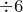in disguise, so we do the opposite, which isto both sides.and remember to expand the brackets correctly — multiplying both internal termsNow what’s the most tangling aspect of our equation? The fact that there is anon both sides of the equals. That is easy to fix. On the right hand side we are subtracting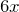, so we do the opposite, which is to add, and watch the equation untangle further. Theterm on the right hand side disappears completely, and the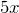we had on the left hand side is increased byto.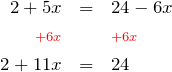This is looking much simpler, but there’s still a tangle of numbers and pronumerals on the left, so let’s focus on that and get rid of the 2 by subtracting.and now all we need to do is the opposite of the multiplication by 11, which is, of course, dividing by 11, and we have solved our equation.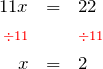And there you have it. We’ve solved quite a complicated equation, and although it did take a number of steps, every step was essentially the same — a direct application of our simple untangling process. At this point it is always worth a quick substitution ofto check the solution. First the left hand side:and then the right hand side: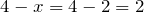Since these are equal, we can be sure our solution is correct.

## What have we learned?

By looking at equations in this manner, we have learned that we do not need to focus on the different types of equation with special rules for each, but can simply repeat the same 2 step process whatever the type:

1. Untangle the expressions by using the opposite operator,
2. Whatever you do to one side, you must do the exact same thing to the other.

Now practice your skills by generating and interactively solving equations and inequations of increasing complexity with the Equation Solver activity at the Mathenæum.

## Prepare to be bamboozled!

Usually errors in calculating the solution to an equation arise because of oversight — forgetting to apply the untangling operation to both sides, or forgetting to multiply both terms inside a bracket for example. However, in the following bamboozler, the solution appears unaffected by such errors. Can you explain why?

#### Bamboozler: A very friendly equation

Imagine the following equation was set as an exam question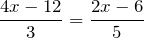One student followed our solution process and wrote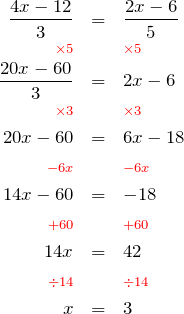which is indeed correct.

Another student started well, multiplying by 5, but then just forgot about the 3 in the denominator, and wrotewhich is the correct answer again. Strange…

Another student made a similar error, multiplying by 3, but forgetting about the 5 in the denominator, and wrotewhich is also the correct answer. Stranger and stranger…

Yet another student just forgot about the denominators entirely, and wrote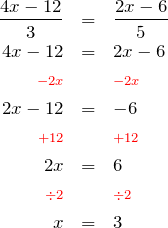which is once again the correct answer.

Now that’s just too weird! It certainly seems a very friendly equation — one that defies all kinds of efforts to get it wrong.

Can you explain what is going on?Mr Wessen

Sometime teacher, sometime programmer, full time mathematician.